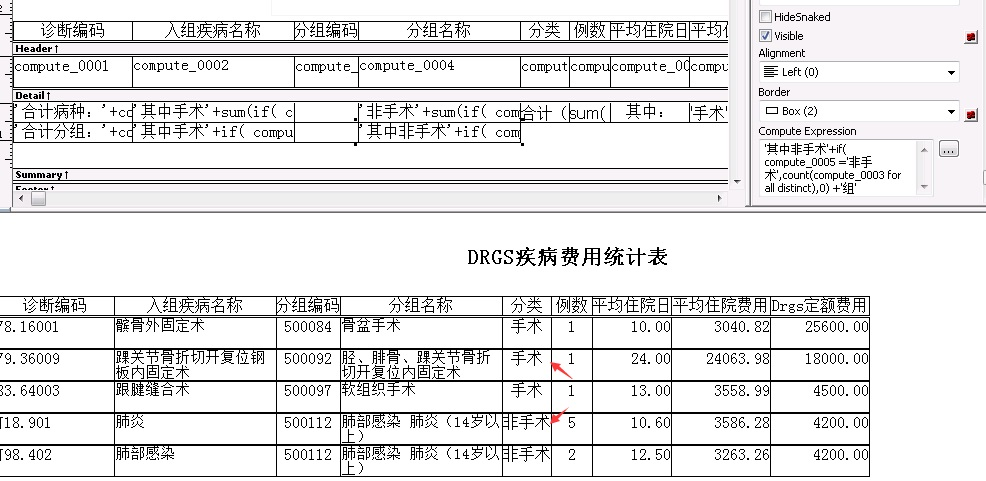数据窗口中统计出满足条件且排除重复记录 条数'其中手术'+if( compute_0005 ='手术',count(compute_0003 for all distinct),0) +'组'
'其中非手术'+if( compute_0005 ='非手术',count(compute_0003 for all distinct),0) +'组'

...全文
544 8 点赞 打赏 收藏 举报

8 条回复

ashinhao 2017-12-07

[quote=引用 3 楼 qq_18219519 的回复:] [quote=引用 1 楼 acen_chen 的回复:] sum(if(compute_0005 ='手术',1,0))

• 打赏
• 举报

[quote=引用 1 楼 acen_chen 的回复:] sum(if(compute_0005 ='手术',1,0))

• 打赏
• 举报

acen_chen 2017-12-06

• 打赏
• 举报

ashinhao 2017-12-05

sum(if(compute_0005 ='手术',1,0))

• 打赏
• 举报

• 打赏
• 举报

sum(if(compute_0005 ='手术',1,0))

• 打赏
• 举报

whb00120012 2017-11-30

• 打赏
• 举报

acen_chen 2017-11-28
sum(if(compute_0005 ='手术',1,0))
• 打赏
• 举报

921

PowerBuilder 相关问题讨论